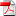# Day-ahead probabilistic forecast of solar irradiance: a Stochastic Differential Equation approach - J. Badosa, E. Gobet, M. Grangereau and D. Kim

17
Jan

In this work, we derive a probabilistic forecast of the solar irradiance
during a day at a given location, using a stochastic differential equation (SDE for short) model. We propose a procedure that transforms a deterministic forecast into a probabilistic forecast: the input parameters of the SDE model are the AROME numerical weather predictions computed at day D-1 for the day D. The model also accounts for the maximal irradiance from the clear sky model. The SDE model is mean-reverting towards the deterministic forecast and the instantaneous amplitude of the noise depends on the clear sky index, so that the fluctuations vanish as the
index is close to 0 (cloudy) or 1 (sunny), as observed in practice. Our tests show a good adequacy of the confidence intervals of the model with the measurement.

•RR-FIME-18-08